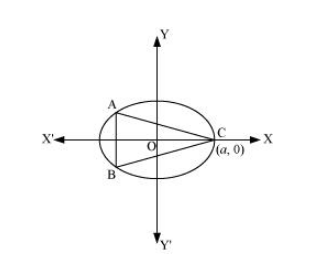# Find the maximum area of an isosceles triangle inscribed in the ellipse

Question:

Find the maximum area of an isosceles triangle inscribed in the ellipse $\frac{x^{2}}{a^{2}}+\frac{y^{2}}{b^{2}}=1$ with its vertex at one end of the major axis.

Solution:The given ellipse is $\frac{x^{2}}{a^{2}}+\frac{y^{2}}{b^{2}}=1$.

Let the major axis be along the x −axis.

Let ABC be the triangle inscribed in the ellipse where vertex C is at (a, 0).

Since the ellipse is symmetrical with respect to the x−axis and y −axis, we can assume the coordinates of A to be (−x1y1) and the coordinates of B to be (−x1, −y1).

Now, we have $y_{1}=\pm \frac{b}{a} \sqrt{a^{2}-x_{1}^{2}}$.

$\therefore$ Coordinates of $\mathrm{A}$ are $\left(-x_{1}, \frac{b}{a} \sqrt{a^{2}-x_{1}^{2}}\right)$ and the coordinates of $\mathrm{B}$ are $\left(x_{1},-\frac{b}{a} \sqrt{a^{2}-x_{1}^{2}}\right)$.

As the point (x1y1) lies on the ellipse, the area of triangle ABC (A) is given by,

\begin{aligned} A=\frac{1}{2} \mid & a\left(\frac{2 b}{a} \sqrt{a^{2}-x_{1}^{2}}\right)+\left(-x_{1}\right)\left(-\frac{b}{a} \sqrt{a^{2}-x_{1}^{2}}\right)+\left(-x_{1}\right)\left(-\frac{b}{a} \sqrt{a^{2}-x_{1}^{2}}\right) \mid \\ \Rightarrow A &=b \sqrt{a^{2}-x_{1}^{2}}+x_{1} \frac{b}{a} \sqrt{a^{2}-x_{1}^{2}} \\ \therefore \frac{d A}{d x_{1}} &=\frac{-2 x_{1} b}{2 \sqrt{a^{2}-x_{1}^{2}}}+\frac{b}{a} \sqrt{a^{2}-x_{1}^{2}}-\frac{2 b x_{1}^{2}}{a 2 \sqrt{a^{2}-x_{1}^{2}}} \\ &=\frac{b}{a \sqrt{a^{2}-x_{1}^{2}}}\left[-x_{1} a+\left(a^{2}-x_{1}^{2}\right)-x_{1}^{2}\right] \\ &=\frac{b\left(-2 x_{1}^{2}-x_{1} a+a^{2}\right)}{a \sqrt{a^{2}-x_{1}^{2}}} \end{aligned}

Now, $\frac{d A}{d x_{1}}=0$

$\Rightarrow-2 x_{1}^{2}-x_{1} a+a^{2}=0$

$\Rightarrow x_{1}=\frac{a \pm \sqrt{a^{2}-4(-2)\left(a^{2}\right)}}{2(-2)}$

$=\frac{a \pm \sqrt{9 a^{2}}}{-4}$

$=\frac{a \pm 3 a}{-4}$

$\Rightarrow x_{1}=-a, \frac{a}{2}$

But, x1 cannot be equal to a.

$\therefore x_{1}=\frac{a}{2} \Rightarrow y_{1}=\frac{b}{a} \sqrt{a^{2}-\frac{a^{2}}{4}}=\frac{b a}{2 a} \sqrt{3}=\frac{\sqrt{3} b}{2}$

Now, $\frac{d^{2} A}{d x_{1}^{2}}=\frac{b}{a}\left\{\frac{\sqrt{a^{2}-x_{1}^{2}}\left(-4 x_{1}-a\right)-\left(-2 x_{1}^{2}-x_{1} a+a^{2}\right) \frac{\left(-2 x_{1}\right)}{2 \sqrt{a^{2}-x_{1}^{2}}}}{a^{2}-x_{1}^{2}}\right\}$

$=\frac{b}{a}\left\{\frac{\left(a^{2}-x_{1}^{2}\right)\left(-4 x_{1}-a\right)+x_{1}\left(-2 x_{1}^{2}-x_{1} a+a^{2}\right)}{\left(a^{2}-x_{1}^{2}\right)^{\frac{3}{2}}}\right\}$

$=\frac{b}{a}\left\{\frac{2 x^{3}-3 a^{2} x-a^{3}}{\left(a^{2}-x_{1}^{2}\right)^{\frac{3}{2}}}\right\}$

Also, when $x_{1}=\frac{a}{2}$, then

$\frac{d^{2} A}{d x_{1}^{2}}=\frac{b}{a}\left\{\frac{2 \frac{a^{3}}{8}-3 \frac{a^{3}}{2}-a^{3}}{\left(\frac{3 a^{2}}{4}\right)^{\frac{3}{2}}}\right\}=\frac{b}{a}\left\{\frac{\frac{a^{3}}{4}-\frac{3}{2} a^{3}-a^{3}}{\left(\frac{3 a^{2}}{4}\right)^{\frac{3}{2}}}\right\}$

$=-\frac{b}{a}\left\{\frac{\frac{9}{4} a^{3}}{\left(\frac{3 a^{2}}{4}\right)^{\frac{3}{2}}}\right\}<0$

Thus, the area is the maximum when $x_{1}=\frac{a}{2}$.

∴ Maximum area of the triangle is given by,

\begin{aligned} A &=b \sqrt{a^{2}-\frac{a^{2}}{4}}+\left(\frac{a}{2}\right) \frac{b}{a} \sqrt{a^{2}-\frac{a^{2}}{4}} \\ &=a b \frac{\sqrt{3}}{2}+\left(\frac{a}{2}\right) \frac{b}{a} \times \frac{a \sqrt{3}}{2} \\ &=\frac{a b \sqrt{3}}{2}+\frac{a b \sqrt{3}}{4}=\frac{3 \sqrt{3}}{4} a b \end{aligned}